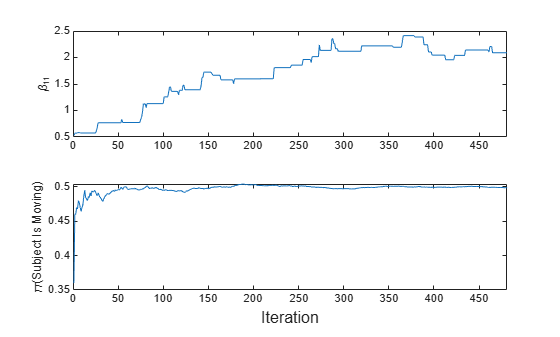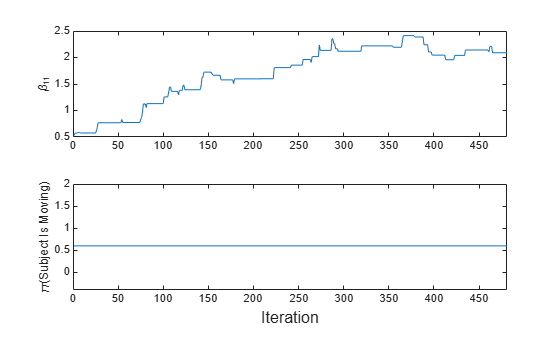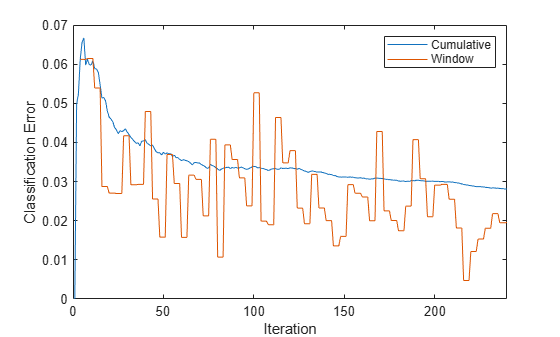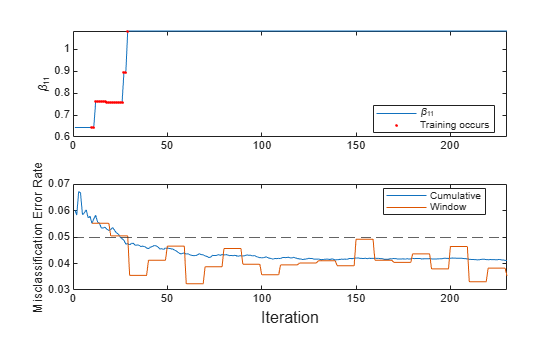# fit

Train ECOC classification model for incremental learning

Since R2022a

## Syntax

Mdl = fit(Mdl,X,Y)
Mdl = fit(Mdl,X,Y,Name=Value)

## Description

The fit function fits a configured multiclass error-correcting output codes (ECOC) classification model for incremental learning (incrementalClassificationECOC object) to streaming data. To additionally track performance metrics using the data as it arrives, use updateMetricsAndFit instead.

To fit or cross-validate an ECOC classification model to an entire batch of data at once, see fitcecoc.

example

Mdl = fit(Mdl,X,Y) returns an incremental learning model Mdl, which represents the input incremental learning model Mdl trained using the predictor and response data, X and Y respectively. Specifically, fit fits the model to the incoming data and stores the updated binary learners and configurations in the output model Mdl.

example

Mdl = fit(Mdl,X,Y,Name=Value) uses additional options specified by one or more name-value arguments. For example, you can specify that the columns of the predictor data matrix correspond to observations, and set observation weights.

## Examples

collapse all

Fit an incremental ECOC learner when you know only the expected maximum number of classes in the data.

Create an incremental ECOC model. Specify that the maximum number of expected classes is 5.

Mdl = incrementalClassificationECOC(MaxNumClasses=5)
Mdl = incrementalClassificationECOC IsWarm: 0 Metrics: [1x2 table] ClassNames: [1x0 double] ScoreTransform: 'none' BinaryLearners: {10x1 cell} CodingName: 'onevsone' Decoding: 'lossweighted' Properties, Methods 

Mdl is an incrementalClassificationECOC model. All its properties are read-only. Mdl can process at most 5 unique classes. By default, the prior class distribution Mdl.Prior is empirical, which means the software updates the prior distribution as it encounters labels.

Mdl must be fit to data before you can use it to perform any other operations.

Load the human activity data set. Randomly shuffle the data.

load humanactivity n = numel(actid); rng(1) % For reproducibility idx = randsample(n,n); X = feat(idx,:); Y = actid(idx);

For details on the data set, enter Description at the command line.

Fit the incremental model to the training data, in chunks of 50 observations at a time, by using the fit function. At each iteration:

• Simulate a data stream by processing 50 observations.

• Overwrite the previous incremental model with a new one fitted to the incoming observations.

• Store the first model coefficient of the first binary learner ${\beta }_{11}$ and the prior probability that the subject is moving (Y > 2) to see how these parameters evolve during incremental learning.

% Preallocation numObsPerChunk = 50; nchunk = floor(n/numObsPerChunk); beta11 = zeros(nchunk,1); priormoved = zeros(nchunk,1); % Incremental fitting for j = 1:nchunk ibegin = min(n,numObsPerChunk*(j-1) + 1); iend = min(n,numObsPerChunk*j); idx = ibegin:iend; Mdl = fit(Mdl,X(idx,:),Y(idx)); beta11(j) = Mdl.BinaryLearners{1}.Beta(1); priormoved(j) = sum(Mdl.Prior(Mdl.ClassNames > 2)); end

Mdl is an incrementalClassificationECOC model object trained on all the data in the stream.

To see how the parameters evolve during incremental learning, plot them on separate tiles.

t = tiledlayout(2,1); nexttile plot(beta11) xlim([0 nchunk]) ylabel("\beta_{11}") nexttile plot(priormoved) xlim([0 nchunk]) ylabel("\pi(Subject Is Moving)") xlabel(t,"Iteration")fit updates the coefficient as it processes each chunk. Because the prior class distribution is empirical, $\pi$(subject is moving) changes as fit processes each chunk.

Fit an incremental ECOC learner when you know all the class names in the data.

Consider training a device to predict whether a subject is sitting, standing, walking, running, or dancing based on biometric data measured on the subject. The class names map 1 through 5 to an activity. Also, suppose that the researchers plan to expose the device to each class uniformly.

Create an incremental ECOC model for multiclass learning. Specify the class names and the uniform prior class distribution.

classnames = 1:5; Mdl = incrementalClassificationECOC(ClassNames=classnames,Prior="uniform")
Mdl = incrementalClassificationECOC IsWarm: 0 Metrics: [1x2 table] ClassNames: [1 2 3 4 5] ScoreTransform: 'none' BinaryLearners: {10x1 cell} CodingName: 'onevsone' Decoding: 'lossweighted' Properties, Methods 

Mdl is an incrementalClassificationECOC model object. All its properties are read-only. During training, observed labels must be in Mdl.ClassNames.

Mdl must be fit to data before you can use it to perform any other operations.

Load the human activity data set. Randomly shuffle the data.

load humanactivity n = numel(actid); rng(1) % For reproducibility idx = randsample(n,n); X = feat(idx,:); Y = actid(idx);

For details on the data set, enter Description at the command line.

Fit the incremental model to the training data by using the fit function. Simulate a data stream by processing chunks of 50 observations at a time. At each iteration:

• Process 50 observations.

• Overwrite the previous incremental model with a new one fitted to the incoming observations.

• Store the first model coefficient of the first binary learner ${\beta }_{11}$ and the prior probability that the subject is moving (Y > 2) to see how these parameters evolve during incremental learning.

% Preallocation numObsPerChunk = 50; nchunk = floor(n/numObsPerChunk); beta11 = zeros(nchunk,1); priormoved = zeros(nchunk,1); % Incremental fitting for j = 1:nchunk ibegin = min(n,numObsPerChunk*(j-1) + 1); iend = min(n,numObsPerChunk*j); idx = ibegin:iend; Mdl = fit(Mdl,X(idx,:),Y(idx)); beta11(j) = Mdl.BinaryLearners{1}.Beta(1); priormoved(j) = sum(Mdl.Prior(Mdl.ClassNames > 2)); end

Mdl is an incrementalClassificationECOC model object trained on all the data in the stream.

To see how the parameters evolve during incremental learning, plot them on separate tiles.

t = tiledlayout(2,1); nexttile plot(beta11) xlim([0 nchunk]) ylabel("\beta_{11}") nexttile plot(priormoved) xlim([0 nchunk]) ylabel("\pi(Subject Is Moving)") xlabel(t,"Iteration")fit updates the posterior mean of the predictor distribution as it processes each chunk. Because the prior class distribution is specified as uniform, $\pi$(subject is moving) = 0.6 and does not change as fit processes each chunk.

Train an ECOC classification model by using fitcecoc, convert it to an incremental learner, track its performance on streaming data, and then fit the model to the data. For incremental learning functions, orient the observations in columns, and specify observation weights.

Load the human activity data set. Randomly shuffle the data.

load humanactivity rng(1); % For reproducibility n = numel(actid); idx = randsample(n,n); X = feat(idx,:); Y = actid(idx);

For details on the data set, enter Description at the command line.

Suppose that the data from a stationary subject (Y <= 2) has double the quality of the data from a moving subject. Create a weight variable that assigns a weight of 2 to observations from a stationary subject and 1 to a moving subject.

W = ones(n,1) + (Y <=2);

Train ECOC Classification Model

Fit an ECOC classification model to a random sample of half the data. Specify observation weights.

idxtt = randsample([true false],n,true); TTMdl = fitcecoc(X(idxtt,:),Y(idxtt),Weights=W(idxtt))
TTMdl = ClassificationECOC ResponseName: 'Y' CategoricalPredictors: [] ClassNames: [1 2 3 4 5] ScoreTransform: 'none' BinaryLearners: {10x1 cell} CodingName: 'onevsone' Properties, Methods 

TTMdl is a ClassificationECOC model object representing a traditionally trained ECOC classification model.

Convert Trained Model

Convert the traditionally trained model to a model for incremental learning.

IncrementalMdl = incrementalLearner(TTMdl)
IncrementalMdl = incrementalClassificationECOC IsWarm: 1 Metrics: [1x2 table] ClassNames: [1 2 3 4 5] ScoreTransform: 'none' BinaryLearners: {10x1 cell} CodingName: 'onevsone' Decoding: 'lossweighted' Properties, Methods 

IncrementalMdl is an incrementalClassificationECOC model. Because class names are specified in IncrementalMdl.ClassNames, labels encountered during incremental learning must be in IncrementalMdl.ClassNames.

Separately Track Performance Metrics and Fit Model

Perform incremental learning on the rest of the data by using the updateMetrics and fit functions. For incremental learning, orient the observations of the predictor data in columns. At each iteration:

1. Simulate a data stream by processing 50 observations at a time.

2. Call updateMetrics to update the cumulative and window classification error of the model given the incoming chunk of observations. Overwrite the previous incremental model to update the losses in the Metrics property. Note that the function does not fit the model to the chunk of data—the chunk is "new" data for the model. Specify that the observations are oriented in columns, and specify the observation weights.

3. Store the classification error.

4. Call fit to fit the model to the incoming chunk of observations. Overwrite the previous incremental model to update the model parameters. Specify that the observations are oriented in columns, and specify the observation weights.

% Preallocation idxil = ~idxtt; nil = sum(idxil); numObsPerChunk = 50; nchunk = floor(nil/numObsPerChunk); mc = array2table(zeros(nchunk,2),VariableNames=["Cumulative","Window"]); Xil = X(idxil,:)'; Yil = Y(idxil); Wil = W(idxil); % Incremental fitting for j = 1:nchunk ibegin = min(nil,numObsPerChunk*(j-1) + 1); iend = min(nil,numObsPerChunk*j); idx = ibegin:iend; IncrementalMdl = updateMetrics(IncrementalMdl,Xil(:,idx),Yil(idx), ... Weights=Wil(idx),ObservationsIn="columns"); mc{j,:} = IncrementalMdl.Metrics{"ClassificationError",:}; IncrementalMdl = fit(IncrementalMdl,Xil(:,idx),Yil(idx), ... Weights=Wil(idx),ObservationsIn="columns"); end

IncrementalMdl is an incrementalClassificationECOC model object trained on all the data in the stream.

Alternatively, you can use updateMetricsAndFit to update performance metrics of the model given a new chunk of data, and then fit the model to the data.

Plot a trace plot of the performance metrics.

plot(mc.Variables) xlim([0 nchunk]) legend(mc.Properties.VariableNames) ylabel("Classification Error") xlabel("Iteration")The cumulative loss gradually stabilizes, whereas the window loss jumps throughout the training.

Incrementally train an ECOC classification model only when its performance degrades.

Load the human activity data set. Randomly shuffle the data.

load humanactivity n = numel(actid); rng(1) % For reproducibility idx = randsample(n,n); X = feat(idx,:); Y = actid(idx);

For details on the data set, enter Description at the command line.

Configure an ECOC classification model for incremental learning so that the maximum number of expected classes is 5, and the metrics window size is 1000. Prepare the model for updateMetrics by fitting the model to the first 1000 observations.

Mdl = incrementalClassificationECOC(MaxNumClasses=5,MetricsWindowSize=1000); initobs = 1000; Mdl = fit(Mdl,X(1:initobs,:),Y(1:initobs));

Mdl is an incrementalClassificationECOC model object.

Determine whether the model is warm by querying the model property.

isWarm = Mdl.IsWarm
isWarm = logical 1 

Mdl.IsWarm is 1; therefore, Mdl is warm.

Perform incremental learning, with conditional fitting, by following this procedure for each iteration:

• Simulate a data stream by processing a chunk of 100 observations at a time.

• Update the model performance on the incoming chunk of data.

• Fit the model to the chunk of data only when the misclassification error rate is greater than 0.05.

• When tracking performance and fitting, overwrite the previous incremental model.

• Store the misclassification error rate and the first model coefficient of the first binary learner ${\beta }_{11}$ to see how they evolve during training.

• Track when fit trains the model.

% Preallocation numObsPerChunk = 100; nchunk = floor((n - initobs)/numObsPerChunk); beta11 = zeros(nchunk,1); ce = array2table(nan(nchunk,2),VariableNames=["Cumulative","Window"]); trained = false(nchunk,1); % Incremental fitting for j = 1:nchunk ibegin = min(n,numObsPerChunk*(j-1) + 1 + initobs); iend = min(n,numObsPerChunk*j + initobs); idx = ibegin:iend; Mdl = updateMetrics(Mdl,X(idx,:),Y(idx)); ce{j,:} = Mdl.Metrics{"ClassificationError",:}; if ce{j,2} > 0.05 Mdl = fit(Mdl,X(idx,:),Y(idx)); trained(j) = true; end beta11(j) = Mdl.BinaryLearners{1}.Beta(1); end

Mdl is an incrementalClassificationECOC model object trained on all the data in the stream.

To see how the model performance and ${\beta }_{11}$ evolve during training, plot them on separate tiles.

t = tiledlayout(2,1); nexttile plot(beta11) hold on plot(find(trained),beta11(trained),"r.") xlim([0 nchunk]) ylabel("\beta_{11}") legend("\beta_{11}","Training occurs",Location="best") hold off nexttile plot(ce.Variables) yline(0.05,"--") xlim([0 nchunk]) ylabel("Misclassification Error Rate") legend(ce.Properties.VariableNames,Location="best") xlabel(t,"Iteration")The trace plot of ${\beta }_{11}$ shows periods of constant values, during which the loss within the previous observation window is at most 0.05.

## Input Arguments

collapse all

Incremental learning model to fit to streaming data, specified as an incrementalClassificationECOC model object. You can create Mdl by calling incrementalClassificationECOC directly, or by converting a supported, traditionally trained machine learning model using the incrementalLearner function.

Chunk of predictor data, specified as a floating-point matrix of n observations and Mdl.NumPredictors predictor variables. The value of the ObservationsIn name-value argument determines the orientation of the variables and observations. The default ObservationsIn value is "rows", which indicates that observations in the predictor data are oriented along the rows of X.

The length of the observation labels Y and the number of observations in X must be equal; Y(j) is the label of observation j (row or column) in X.

Note

• If Mdl.NumPredictors = 0, fit infers the number of predictors from X, and sets the corresponding property of the output model. Otherwise, if the number of predictor variables in the streaming data changes from Mdl.NumPredictors, fit issues an error.

• fit supports only floating-point input predictor data. If your input data includes categorical data, you must prepare an encoded version of the categorical data. Use dummyvar to convert each categorical variable to a numeric matrix of dummy variables. Then, concatenate all dummy variable matrices and any other numeric predictors. For more details, see Dummy Variables.

Data Types: single | double

Chunk of labels, specified as a categorical, character, or string array, a logical or floating-point vector, or a cell array of character vectors.

The length of the observation labels Y and the number of observations in X must be equal; Y(j) is the label of observation j (row or column) in X.

fit issues an error when one or both of these conditions are met:

• Y contains a new label and the maximum number of classes has already been reached (see the MaxNumClasses and ClassNames arguments of incrementalClassificationECOC).

• The ClassNames property of the input model Mdl is nonempty, and the data types of Y and Mdl.ClassNames are different.

Data Types: char | string | cell | categorical | logical | single | double

Note

If an observation (predictor or label) or weight contains at least one missing (NaN) value, fit ignores the observation. Consequently, fit uses fewer than n observations to create an updated model, where n is the number of observations in X.

### Name-Value Arguments

Specify optional pairs of arguments as Name1=Value1,...,NameN=ValueN, where Name is the argument name and Value is the corresponding value. Name-value arguments must appear after other arguments, but the order of the pairs does not matter.

Example: ObservationsIn="columns",Weights=W specifies that the columns of the predictor matrix correspond to observations, and the vector W contains observation weights to apply during incremental learning.

Predictor data observation dimension, specified as "rows" or "columns".

Example: ObservationsIn="columns"

Data Types: char | string

Chunk of observation weights, specified as a floating-point vector of positive values. fit weighs the observations in X with the corresponding values in Weights. The size of Weights must equal n, which is the number of observations in X.

By default, Weights is ones(n,1).

For more details, including normalization schemes, see Observation Weights.

Example: Weights=W specifies the observation weights as the vector W.

Data Types: double | single

## Output Arguments

collapse all

Updated ECOC classification model for incremental learning, returned as an incremental learning model object of the same data type as the input model Mdl, an incrementalClassificationECOC object.

If you do not specify all expected classes by using the ClassNames name-value argument when you create the input model Mdl using incrementalClassificationECOC, and Y contains expected, but unprocessed, classes, then fit performs the following actions:

1. Append any new labels in Y to the tail of Mdl.ClassNames.

2. Expand Mdl.Prior to a length c vector of an updated empirical class distribution, where c is the number of classes in Mdl.ClassNames.

## Tips

• Unlike traditional training, incremental learning might not have a separate test (holdout) set. Therefore, to treat each incoming chunk of data as a test set, pass the incremental model and each incoming chunk to updateMetrics before training the model on the same data.

## Algorithms

collapse all

### Observation Weights

If the prior class probability distribution is known (in other words, the prior distribution is not empirical), fit normalizes observation weights to sum to the prior class probabilities in the respective classes. This action implies that the default observation weights are the respective prior class probabilities.

If the prior class probability distribution is empirical, the software normalizes the specified observation weights to sum to 1 each time you call fit.

## Version History

Introduced in R2022a